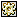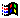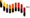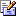Contents Articles Behaviors Books Director News Director Web Sites FAQ Games Mailing Lists News Groups Project Examples Reviews Software Tools Useful Web Sites Utilities Xtras

 Don't miss these#Vigenere cipher (Encryption)

 Compatibilities:Rating:Author: StuartAcklam (website)

This script uses the simple princip from the Vigenere cipher (Encryption)

 --This script is a parent script, this means you need to birth the script before using any handlers/methods within --the code: --gCipherObj = new(script "p: cipher")   -- ("p: cipher" being the name of the parent script cast member) --To get the crypted or the encrypted text: --outPutText = mEnCodeDeCode(object, "input text", "keyword",TRUE/FALSE: crypt/decrypt) --an example: (ENCYPT) --outPutTxt = mEnCodeDeCode(gCipherObj, "Your text must be here", "LURID",0) --"Your text must be here" is encypt(0) with the keyword "LURID". the result is "JILZ WPRK UXDN SM KPLV" --an example: (DECYPT) --outPutTxt = mEnCodeDeCode(gCipherObj, "JILZ WPRK UXDN SM KPLV", "LURID",1) --"JILZ WPRK UXDN SM KPLV" is DEcypted(1) with the keyword "LURID". the result is "YOUR TEXT MUST BE HERE" --| lingoist: s.m.ack stuart m. acklam, 27th June 2000, Dir 8.0 --| http://www.acklam.com -- mailto:stuart@acklam.com --| --| Version History: --| 000627: lingo / demo done s.m.ack --| --| --|------------------ DISCRIPTION -------------------- --| This script uses the simple princip from the Vigenere cipher. --| The Vigenere cipher is a simple example of a polyalphabetic cipher. --| In its original form, it is based on the Vigenere tableau (see the method mSetUpAlphaBet) --| To encode using this cipher, first choose a keyword, such as "LURID", and write it above the plain text repeatedly: --|             LURI DL URIDLURI DL URI DLU RID LURI      - "LURID" --| Plain Text: MEET AT MIDNIGHT BY THE OLD OAK TREE --| The cipher letter is the letter in the tableau determined by the keyword column and the plaintext row. --| Thus the first "M" of the message is encrypted as "X", the first "E" as "Y", and so on. --| To decrypt the Vigenere cipher, you run this process in reverse. Alternatively, --| you can reverse the keyword by replacing every letter by its negative (mod 26). --| For example, LURID contains letters numbered 11,20,17,8, and 3 (with A=0). --| The negatives mod 26 are 26-11=15, 26-20=6, 26-17=9,26-8=18, and 26-3=23, which --| corresponds to the word PGJSX. So encrypting with PGJSX decrypts LURID. --| --| more infomation can be found on the Vigenere cipher via http://raphael.math.uic.edu/~jeremy/crypt/vignere.html --| --| --|------------------ HOW TO USE THIS SCRIPT -------------------- --| This script is a parent script, this means you need to birth the script before using any handlers/methods within the code: --| gCipherObj = new(script "p: cipher")   -- ("p: cipher" being the name of the parent script cast member) --| --| To get the crypted or the encrypted text: --| outPutText = mEnCodeDeCode(object, "input text", "keyword",TRUE/FALSE: crypt/decrypt) --| --| an example: (ENCYPT) --| outPutTxt = mEnCodeDeCode(gCipherObj, "Your text must be here", "LURID", 0) --| "Your text must be here" is encypt(0) with the keyword "LURID". the result is "JILZ WPRK UXDN SM KPLV" --| --| an example: (DECYPT) --| outPutTxt = mEnCodeDeCode(gCipherObj, "JILZ WPRK UXDN SM KPLV", "LURID", 1) --| "JILZ WPRK UXDN SM KPLV" is DEcypted(1) with the keyword "LURID". the result is "YOUR TEXT MUST BE HERE" ----------------------------------------------------------- ----------------------------------------------------------- property pAlphabetList   -- contains the Vigenere Tableau on new me   pAlphabetList = []   mSetUpAlphaBet(me)   return me end ---------------------------------------------------------------------------- --------------------------- -- set up the Vigenere tableau as a lingo list on mSetUpAlphaBet me   pAlphabetList.append(["A", "B", "C", "D", "E", "F", "G", "H", "I", "J", "K", "L", "M", "N", "O", "P", "Q", "R", "S", "T", "U", "V", "W", "X", "Y", "Z"])   pAlphabetList.append(["B", "C", "D", "E", "F", "G", "H", "I", "J", "K", "L", "M", "N", "O", "P", "Q", "R", "S", "T", "U", "V", "W", "X", "Y", "Z", "A"])   pAlphabetList.append(["C", "D", "E", "F", "G", "H", "I", "J", "K", "L","M", "N", "O", "P", "Q", "R", "S", "T", "U", "V", "W", "X", "Y", "Z", "A","B"])   pAlphabetList.append(["D", "E", "F", "G", "H", "I", "J", "K", "L", "M","N", "O", "P", "Q", "R", "S", "T", "U", "V", "W", "X", "Y", "Z", "A", "B","C"])   pAlphabetList.append(["E", "F", "G", "H", "I", "J", "K", "L", "M", "N","O", "P", "Q", "R", "S", "T", "U", "V", "W", "X", "Y", "Z", "A", "B", "C","D"])   pAlphabetList.append(["F", "G", "H", "I", "J", "K", "L", "M", "N", "O","P", "Q", "R", "S", "T", "U", "V", "W", "X", "Y", "Z", "A", "B", "C", "D","E"])   pAlphabetList.append(["G", "H", "I", "J", "K", "L", "M", "N", "O", "P","Q", "R", "S", "T", "U", "V", "W", "X", "Y", "Z", "A", "B", "C", "D", "E","F"])   pAlphabetList.append(["H", "I", "J", "K", "L", "M", "N", "O", "P", "Q","R", "S", "T", "U", "V", "W", "X", "Y", "Z", "A", "B", "C", "D", "E", "F","G"])   pAlphabetList.append(["I", "J", "K", "L", "M", "N", "O", "P", "Q", "R","S", "T", "U", "V", "W", "X", "Y", "Z", "A", "B", "C", "D", "E", "F", "G","H"])   pAlphabetList.append(["J", "K", "L", "M", "N", "O", "P", "Q", "R", "S","T", "U", "V", "W", "X", "Y", "Z", "A", "B", "C", "D", "E", "F", "G", "H","I"])   pAlphabetList.append(["K", "L", "M", "N", "O", "P", "Q", "R", "S", "T","U", "V", "W", "X", "Y", "Z", "A", "B", "C", "D", "E", "F", "G", "H", "I","J"])   pAlphabetList.append(["L", "M", "N", "O", "P", "Q", "R", "S", "T", "U","V", "W", "X", "Y", "Z", "A", "B", "C", "D", "E", "F", "G", "H", "I", "J","K"])   pAlphabetList.append(["M", "N", "O", "P", "Q", "R", "S", "T", "U", "V","W", "X", "Y", "Z", "A", "B", "C", "D", "E", "F", "G", "H", "I", "J", "K","L"])   pAlphabetList.append(["N", "O", "P", "Q", "R", "S", "T", "U", "V", "W","X", "Y", "Z", "A", "B", "C", "D", "E", "F", "G", "H", "I", "J", "K", "L","M"])   pAlphabetList.append(["O", "P", "Q", "R", "S", "T", "U", "V", "W", "X","Y", "Z", "A", "B", "C", "D", "E", "F", "G", "H", "I", "J", "K", "L", "M","N"])   pAlphabetList.append(["P", "Q", "R", "S", "T", "U", "V", "W", "X", "Y","Z", "A", "B", "C", "D", "E", "F", "G", "H", "I", "J", "K", "L", "M", "N","O"])   pAlphabetList.append(["Q", "R", "S", "T", "U", "V", "W", "X", "Y", "Z","A", "B", "C", "D", "E", "F", "G", "H", "I", "J", "K", "L", "M", "N", "O","P"])   pAlphabetList.append(["R", "S", "T", "U", "V", "W", "X", "Y", "Z", "A","B", "C", "D", "E", "F", "G", "H", "I", "J", "K", "L", "M", "N", "O", "P","Q"])   pAlphabetList.append(["S", "T", "U", "V", "W", "X", "Y", "Z", "A", "B","C", "D", "E", "F", "G", "H", "I", "J", "K", "L", "M", "N", "O", "P", "Q","R"])   pAlphabetList.append(["T", "U", "V", "W", "X", "Y", "Z", "A", "B", "C","D", "E", "F", "G", "H", "I", "J", "K", "L", "M", "N", "O", "P", "Q", "R","S"])   pAlphabetList.append(["U", "V", "W", "X", "Y", "Z", "A", "B", "C", "D","E", "F", "G", "H", "I", "J", "K", "L", "M", "N", "O", "P", "Q", "R", "S","T"])   pAlphabetList.append(["V", "W", "X", "Y", "Z", "A", "B", "C", "D", "E","F", "G", "H", "I", "J", "K", "L", "M", "N", "O", "P", "Q", "R", "S", "T","U"])   pAlphabetList.append(["W", "X", "Y", "Z", "A", "B", "C", "D", "E", "F","G", "H", "I", "J", "K", "L", "M", "N", "O", "P", "Q", "R", "S", "T", "U","V"])   pAlphabetList.append(["X", "Y", "Z", "A", "B", "C", "D", "E", "F", "G","H", "I", "J", "K", "L", "M", "N", "O", "P", "Q", "R", "S", "T", "U", "V","W"])   pAlphabetList.append(["Y", "Z", "A", "B", "C", "D", "E", "F", "G", "H","I", "J", "K", "L", "M", "N", "O", "P", "Q", "R", "S", "T", "U", "V", "W","X"])   pAlphabetList.append(["Z", "A", "B", "C", "D", "E", "F", "G", "H", "I","J", "K", "L", "M", "N", "O", "P", "Q", "R", "S", "T", "U", "V", "W", "X","Y"]) end ---------------------------------------------------------------------------- --------------------------- -- set up the KEYWODE LIST on mSetUpKeyWord me, vkey, vDecrypt   vReturn = []   repeat with i = 1 to (vkey).length     vReturn[i] = chars (vkey, i, i)   end repeat   -- if the a request to DECRYT, vDecrypt = TRUE   if vDecrypt then     vReturnDeC = []     repeat with i = 1 to vReturn.count       vLet = vReturn[i]       vA = (pAlphabetList.getOne(vLet))-1       vB = (26 - vA)+1       if vB = 27 then vB = 1       vReturnDeC[i] = pAlphabetList[(vB)]     end repeat     vReturn = vReturnDeC   end if   return vReturn end ---------------------------------------------------------------------------- --------------------------- -- ENCYPT or DECYPT the TEXT with the key on mEnCodeDeCode me, wht, vkey, vDecrypt   theTxt = ""   wht = mForceUppercase (me, wht)   vkey = mForceUppercase (me, vkey)   vKeyWordList = mSetUpKeyWord (me, vkey, vDecrypt)  -- sets the string key into a list   vTheCurrentKeyLetter = 0   repeat with i = 1 to (wht).length     if chars (wht, i, i) = " " then       theTxt = theTxt & " "       next repeat     else       vTheCurrentKeyLetter = vTheCurrentKeyLetter + 1       vTheKeyLNum = (vTheCurrentKeyLetter mod (vKeyWordList.count))       if vTheKeyLNum = 0 then vTheKeyLNum = vKeyWordList.count       vTheKeyLetter = vKeyWordList[(vTheKeyLNum)]       vHori =  pAlphabetList.getOne(vTheKeyLetter)       vtheLetter = chars(wht, i, i)       vVerti = pAlphabetList.getOne(vtheLetter)       theLetter = pAlphabetList[vVerti][vHori]       theTxt = theTxt & theLetter     end if   end repeat   return theTxt end ---------------------------------------------------------------------------- ----------------------------- Forces ANY lowercase letters to uppercase - basic lingo from director 8 help (numToChar()) on mForceUppercase me, input   output = EMPTY   num = length(input)   repeat with i = 1 to num     theASCII = charToNum(input.char[i])     if theASCII = min(max(96, theASCII), 123) then       theASCII = theASCII - 32       if theASCII = min(max(63, theASCII), 91) then         put numToChar(theASCII) after output       end if     else       put input.char[i] after output    -- added 0626 (stuart@acklam.com)     end if   end repeat   return output end

 Contact MMI 36 South Court Sq Suite 300 Newnan, GA 30263 USASend e-mail